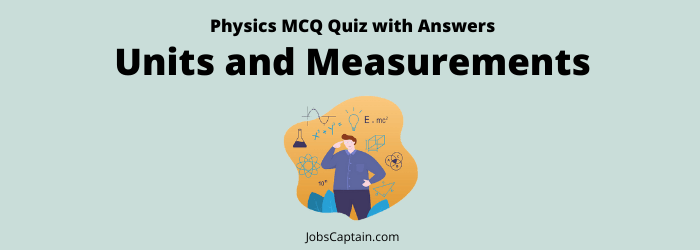# Physics: Units and Measurements MCQ Quiz 1Quiz Set 1 2

Question 1. How can the height of a person who is six feet tall, be expressed (approximately) in nanometre?

(A) 181 × 107 nm

(B) 183 × 107 nm

(C) 234 × 106 nm

(D) 183 × 106 nm

(B) 183 × 107 nm

Question 2. A nanometre is equal to?

(A) 10–9 cm

(B) 10–8 cm

(C) 10–7 cm

(D) 10–6 cm

(C) 10–7 cm

Question 3. Ampere is the unit of?

(A) Power

(B) Resistance

(C) Electric current

(D) Voltage

(C) Electric current

Question 4. Megawatt is the measuring unit of power which is?

(A) Lost in transmission

(B) Saved

(C) Consumed

(D) Generated

(D) Generated

Question 5. Which one of the following SI unit is not correctly matched?

(A) Pressure – Dyne

(B) Mass – kg

(C) Force – Newton

(D) Work – Joule

(A) Pressure – Dyne

Question 6. Which one of the following is not correctly matched?

(A) Light year – Unit of measuring time

(B) Angstrom – Unit of wavelength of light

(C) Nautical mile – Unit of distance used in navigation

(D) Knot – Measure of speed of ship

(A) Light year – Unit of measuring time

Question 7. How many watts are there in a horsepower?

(A) 748

(B) 746

(C) 750

(D) 1000

(B) 746

Question 8. ‘Joule’ is related to energy in the same way as ‘Pascal’ is related to?

(A) Purity

(B) Density

(C) Pressure

(D) Mass

(C) Pressure

Question 9. One micron is equal to?

(A) 1/10,000 mm

(B) 1/1000 mm

(C) 1/100 mm

(D) 1/10 mm

(B) 1/1000 mm

Question 10. One micron represents a length of?

(A) 1 m

(B) 1 mm

(C) 10–4 cm

(D) 10–6 cm

(C) 10–4 cm

Question 11. Which unit of measurement is multiplied by 0.39 to convert it to ‘inches’?

(A) Decimetre

(B) Metre

(C) Centimetre

(D) Millimetre

(C) Centimetre

Question 12. PARSEC is the unit of?

(A) Magnetic force

(B) Light intensity

(C) Time

(D) Distance

(D) Distance

Question 13. A parsec, a unit of distance used to measure the distance related to the stars in the sky, is equal to?

(A) 3.05 light years

(B) 4.50 light years

(C) 3.25 light years

(D) 4.25 light years

(C) 3.25 light years

Question 14. ‘Light Year’ is?

(A)  The time in which a spacecraft reaches Moon from the Earth

(B) The time which Sun rays take to reach the Earth

(C) The distance travelled by light in one year

(D) The year in which February has 29 days

(C) The distance travelled by light in one year

Question 15. Which one of the following is the unit of measure of the thickness of the ozone layer of the atmosphere?

(A) Maxwell

(B) Poise

(C) Dobson

(D) Knot

(C) Dobson

Question 16. Light year is the unit of?

(A) Intensity of light

(B) Speed of light

(C) Time

(D) Distance

(D) Distance

Question 17. The unit of the force is?

(A) Rutherford

(B) Newton

(C) Fermi

(B) Newton

Question 18. The unit of power is?

(A) Neutrons

(B) Watt

(C) Volts

(D) Hertz

(B) Watt

Question 19. The SI unit of electrical resistance of conductor is?

(A) Ohm-metre

(B) Ampere

(C) Volts

(A) Ohm-metre

Question 20. The unit of work is?

(A) Dyne

(B) Watt

(C) Neutron

(D) Joule